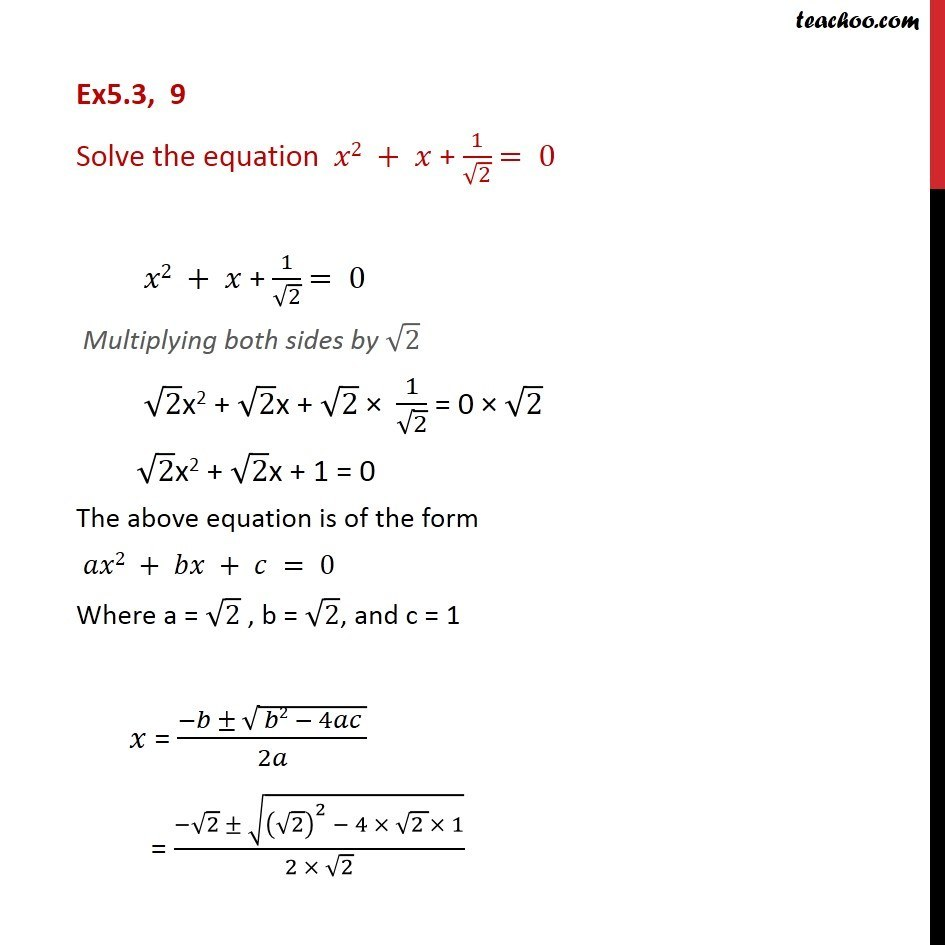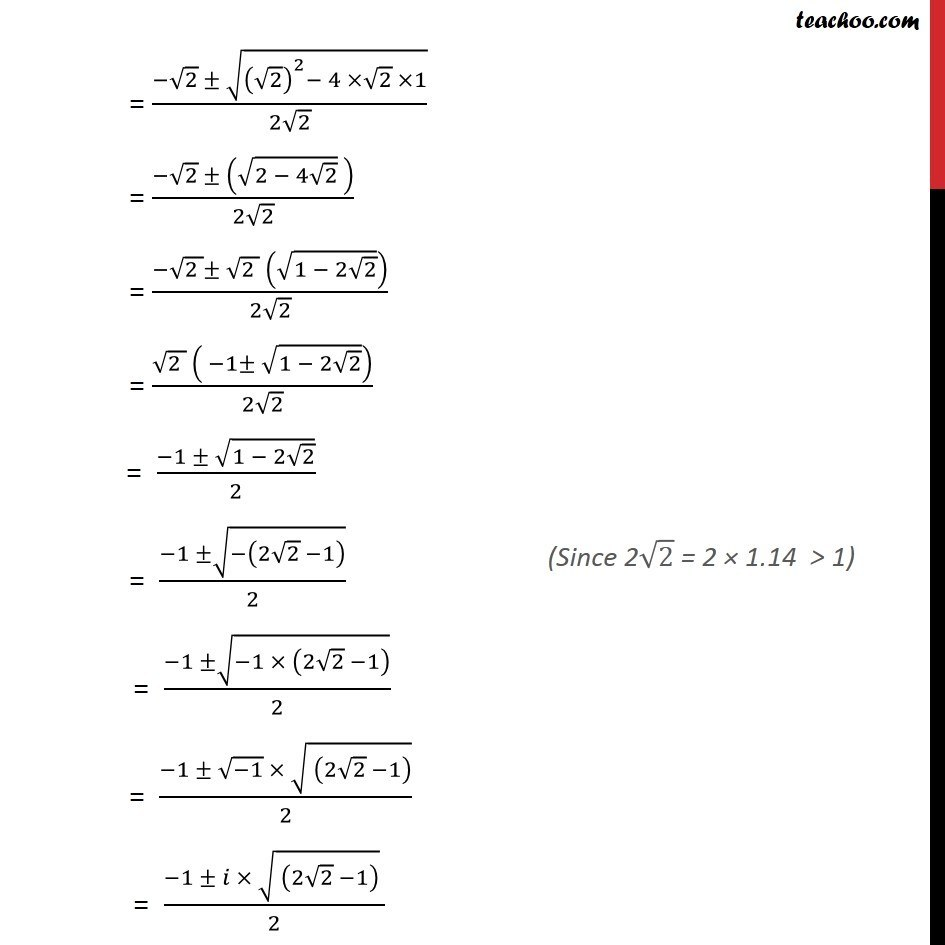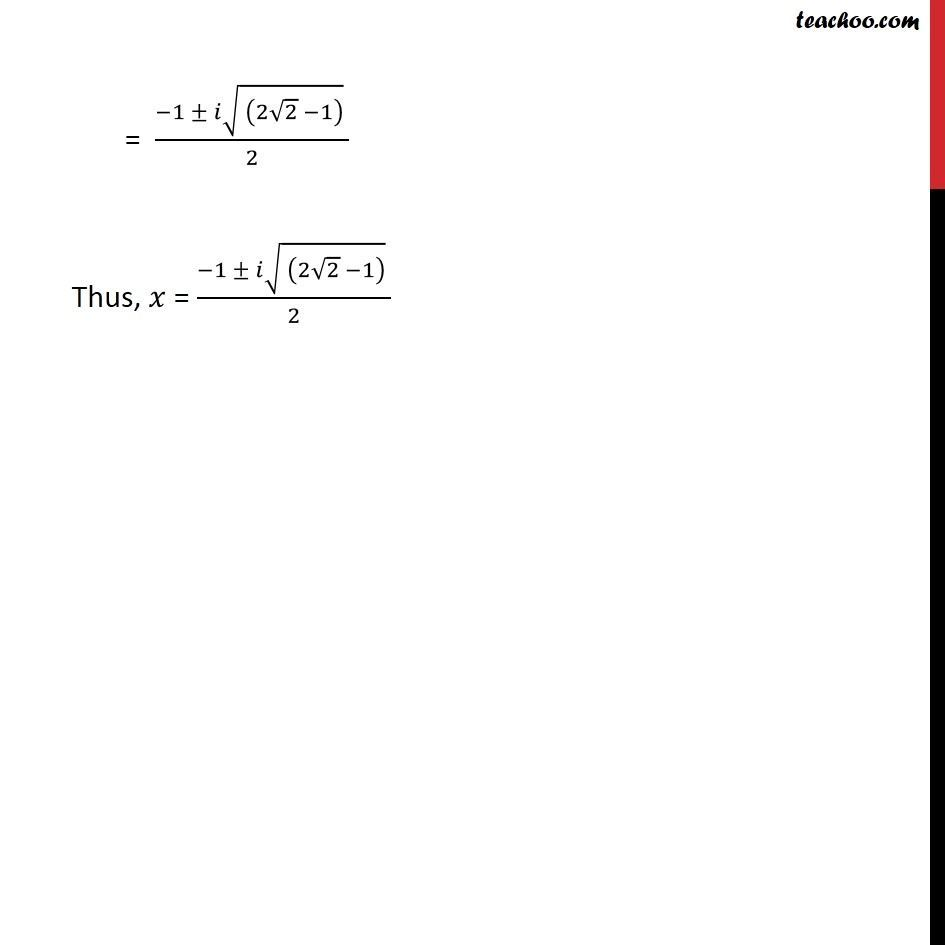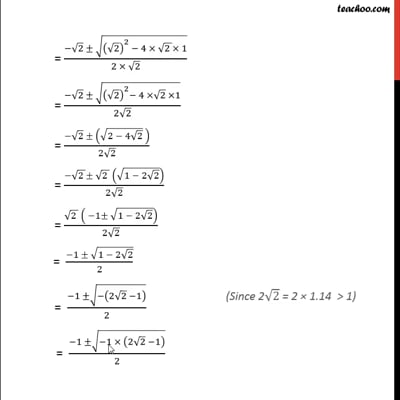Ex 5.3

Chapter 5 Class 11 Complex Numbers (Term 1)
Serial order wiseThis video is only available for Teachoo black users

### Transcript

Ex5.3, 9 Solve the equation 2 + + 1/ 2 = 0 2 + + 1/ 2 = 0 Multiplying both sides by 2 2x2 + 2x + 2 1/ 2 = 0 2 2x2 + 2x + 1 = 0 The above equation is of the form 2 + + = 0 Where a = 2 , b = 2, and c = 1 = ( ( 2 4 ))/2 = ( 2 (( 2)^2 4 (2 ) 1))/(2 2) = ( 2 (( 2)^2 4 2 1))/(2 2) = ( 2 ( (2 4 2) ))/(2 2) = ( (2 ) (2 ) ( (1 2 2) ))/(2 2) = ( (2 ) ( 1 (1 2 2) ))/(2 2) = ( 1 (1 2 2) )/2 = ( 1 ( (2 2 1) ))/2 = ( 1 ( 1 (2 2 1) ))/2 = ( 1 ( 1) ( (2 2 1) ))/2 = ( 1 ( (2 2 1) ) )/2 = ( 1 ( (2 2 1) ) )/2 Thus, = ( 1 ( (2 2 1) ) )/2